• 2017-05-09 16:18:43

若已知运算符之间的优先关系，可按如下步骤构造优先函数：

1、对每个运算符a（包括#在内）令f(a)=g(a)=1

2、如果a⋗b且f(a)<=g(b)令f(a)=g(b)+1

3、如果a⋖b且f(a)>=g(b)令g(b)= f(a)+1

4、如果a≐b而f(a) ≠g(b)，令min{f(a),g(b)}=max{f(a),g(b)}

5、重复2~4，直到过程收敛。如果重复过程中有一个值大于2n，则表明不存在算符优先函数。

import java.util.Scanner;

/**
* Created by redli on 2017/5/2.
*
* 样例输入1
* --------------------------------------------------
请输入终结符（输入#号结束）：
+*()i#
请输入关系矩阵(#号结束)：
><<><>><><<<<+<>>!>!>>!>!#
-----------------------------------------------------
* 样例输入2
* --------------------------------------------------
请输入终结符（输入#号结束）：
abcd#
请输入关系矩阵(#号结束)：
!!>>!!>!<=<!=!=!#
-----------------------------------------------------
*/
public class Floyd {
public static Scanner in = new Scanner(System.in);
public static StringBuffer terminal = new StringBuffer();
public static StringBuffer matrix = new StringBuffer();
public  static void main(String args[]){
//输入
Input input = new Input();
input.inputTerminal();
input.inputMatrix();

//优先函数
PriorityFunction priority_function = new PriorityFunction();
priority_function.makePriorityFunction();
priority_function.createPriorityFunction();
priority_function.outPriorityFunction();
}

private static class Input{
//输入终结符
public void inputTerminal(){
System.out.println("请输入终结符（输入#号结束）：");
String str = in.next();
while(!str.equals("#")){
if(str.substring(str.length()-1).equals("#")){
terminal.append(str.substring(0,str.length()-1));
str = "#";
} else{
terminal.append(str);
str = in.next();
}
}
}

/*
* 输入关系矩阵
* 输入>，<，=，！（表示为空）
* */
public void inputMatrix(){
System.out.println("请输入关系矩阵(#号结束)：");
String str = in.next();
while(!(str.equals("#"))){
if(!(str.substring(str.length()-1).equals("#"))){
matrix.append(str);
str = in.next();
} else{
matrix.append(str.substring(0,str.length()-1));
str = "#";
}
}
}
}

/*
* 优先函数处理类
* */
private static class PriorityFunction{
int num = terminal.length();
int [][] arr = new int[num];
/*
* 构造优先函数，赋初值1
* */
public void makePriorityFunction(){
for(int i=0; i<2; i++){
for(int j=0;j<num;j++){
arr[i][j] = 1;
}
}
}

/*
*
* 生成优先函数
*
*  */
public void createPriorityFunction(){
int k=1;
int terLength = terminal.length();
while(k!=0){
k = 0;
for(int i=0; i<terminal.length();i++){
for(int j=0; j<terminal.length(); j++){
if(Character.toString(matrix.charAt(i*terLength+j)).equals(">") && arr[i]<=arr[j]){
arr[i]=arr[j]+1;
k=1;
} else if(Character.toString(matrix.charAt(i*terLength+j)).equals("<") && arr[i]>=arr[j]){
arr[j]=arr[i]+1;
k=1;
}
}
}
}
}

/*
* 输出优先函数
* */
public void outPriorityFunction(){
for(int k=0; k<terminal.length(); k++){
System.out.print(terminal.charAt(k)+" ");
}
System.out.println();
for(int i=0; i<2; i++){
for(int j=0; j<terminal.length();j++){
System.out.print(arr[i][j]+" ");
}
System.out.print("\n");
}
}
}
}

更多相关内容
• ## 算符优先算法中优先函数的构造

千次阅读 多人点赞 2020-11-04 10:29:09
算符优先分析中“优先函数的简单构造！”

### 文章目录

这里我介绍的是一种简单的方法，不是书本的那个方法。是我后面那个参考资料上面的作者想出来的。因为这个是在知网上面找到的，是1997年的一篇文章。我就是一个总结，然后画几个比较清楚的图而已（因为从知网上下载的的pdf上面的图有点不太清楚）

# 优先函数树形构造法

我们教材上面的就是陈火旺老师的那本，然后方法就是找节点。这个方法虽然简单，但是操作起来的却有些麻烦，因为我们作图的时候，难免就会看不清楚，或者数错。下面介绍的树形构造法就可以避免这个问题，仅仅只需要根据优先矩阵就可以得出正确结果。

# 操作步骤

对于一个优先表先做如下几个步骤（假设优先函数存在）
1 ) 对于f(a)对应行中“a>=b 的所有终结符b , 把g(b)作为f(a) 的子树。
2) 对g(a) 对应列中有b <=a 的. 把f(a) 作为g(b)的子树.
3 ) 对f(a)重做第( 1 ) 步, 对g(b) 重做第( 2 ) 步。
4) 重复出现的f或g , 作记号不再对其执行第( 1) , ( 2) 步
方向图
5) 构造f(a)函数时, 以f(a) 为根结点, 按( 1 ) ,(2 ),( 3),( 4) 执行.
6) 构造g(b)函数时, 以g(b) 为根结点, 按(2 ) ,( 1 ) , ( 3 ) , ( 4 ) 执行.
按照以上5步先画出树，然后查树的节点数（包括根节点，做记号的不查），即可以得到根节点的优先函数值。
但是我觉得这个操作步骤2有点问题。应该是b>=a的时候，就把f(a)作为g(b)的子树。我也自己做了实验，发现这样做才是正确的。不过也可能是我没有理解原作者的意思。反正，就目前看来，我都是按照如果b<=a，就把f(a)作为g(b)的子树。
所以，下面我举例的例子，第(2)步都是按照“b>=a的时候，就把f(a)作为g(b)的子树”这个来做的。

# 举例操作

假如现有一个优先矩阵是这样的：这个例子是《编译原理》陈火旺老师（下面我说的教材，没有特别说明也是指的这本教材）那本书上面的。在90页。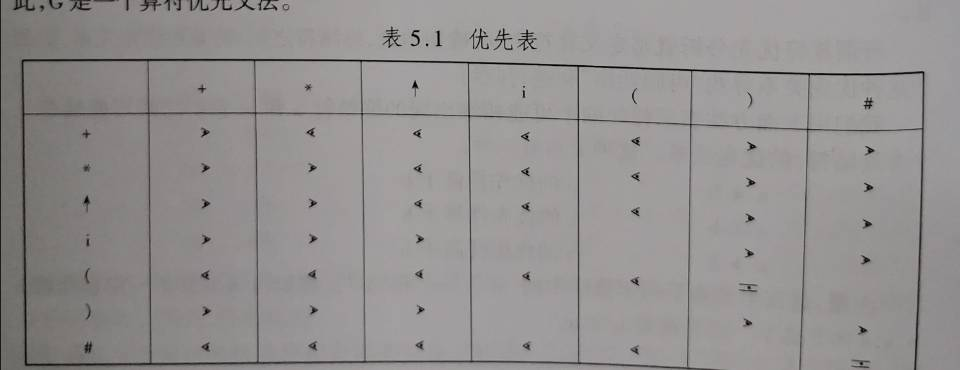那么我们现在用树形构造法来试试怎么得到这个优先函数。因为最后的答案是去掉了 i 和 # 之后得到的，所以我下面也将不会考虑 i 和 # 号。至于为什么不考虑 i 和 #,，就是因为这两个不是算符，我们算符优先函数主要针对的就是算符直接的优先级。但是优先关系矩阵中是给出了 i 和 # 与其他算符之间的优先关系的。我这里其实是有一个疑问的：因为在优先函数这里没有 i 的优先关系，那么在使用优先函数来分析一个句子是不是这个文法定义的合法的句子的时候，那么什么时候该移进这个 i 呢？希望知道的小伙伴可以在下面留言，我们讨论一下。

计算f(+)的优先函数值。

1. 从优先矩阵中可以得到：’+‘ >= 的算符有;’+’,’)’,’#'三个。因为不考虑i 和 #（下面就不提醒这一点了），所以f(+)的孩子节点有两个，分别是g(+)和g()),如下图所示。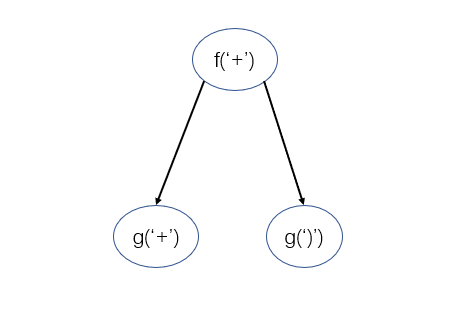2. 现在就来找g(’+’) 的子树。我这里还是采用的是如果b>=a，就把f(a)作为g(b)的子树。从优先表中可以看到的就是’+‘ >= 的算符有：’(’。注意，我这里说的’+‘是g(’+‘)，也就是从’+‘那一列中寻找。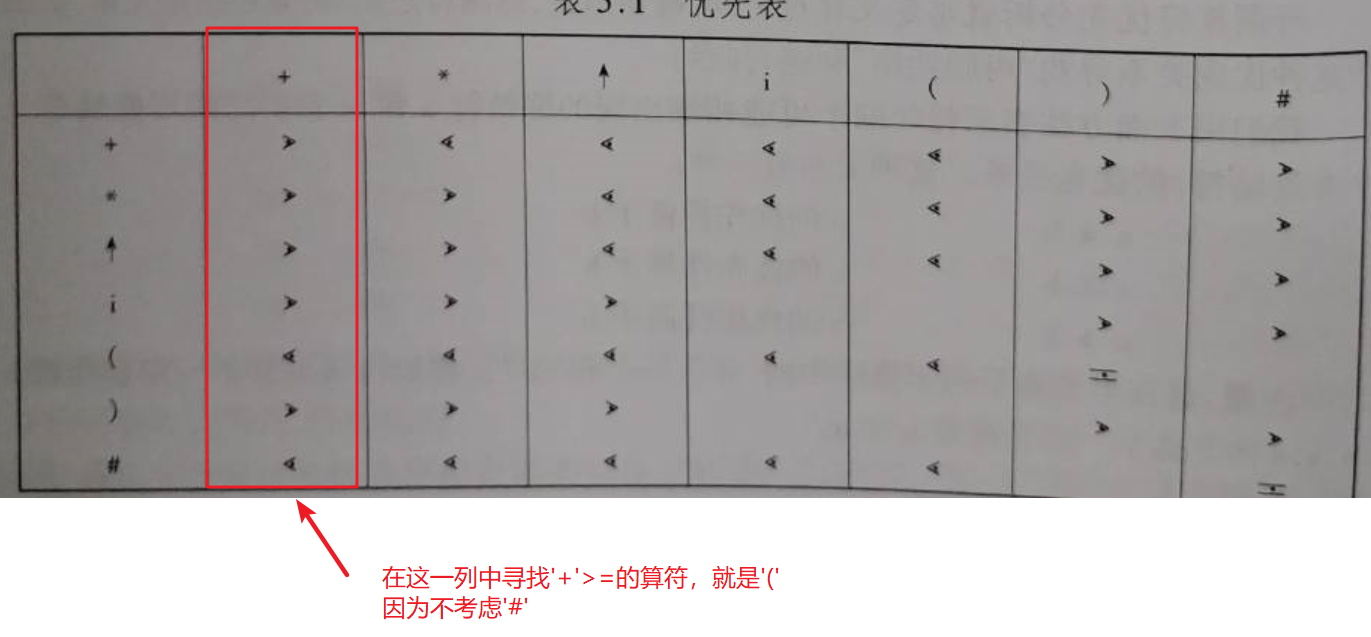所以，g(’+’)的子树就是f(’(’);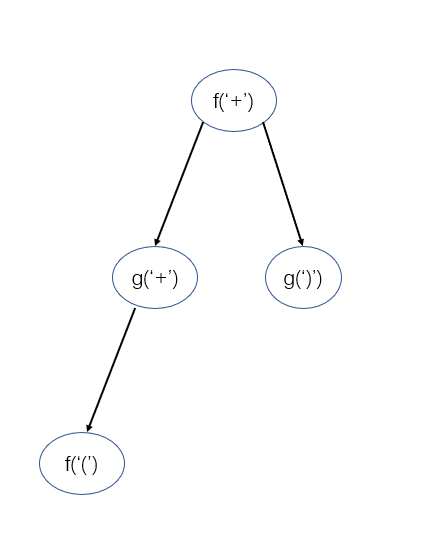3. 然后找到g(’)’) >=的算符：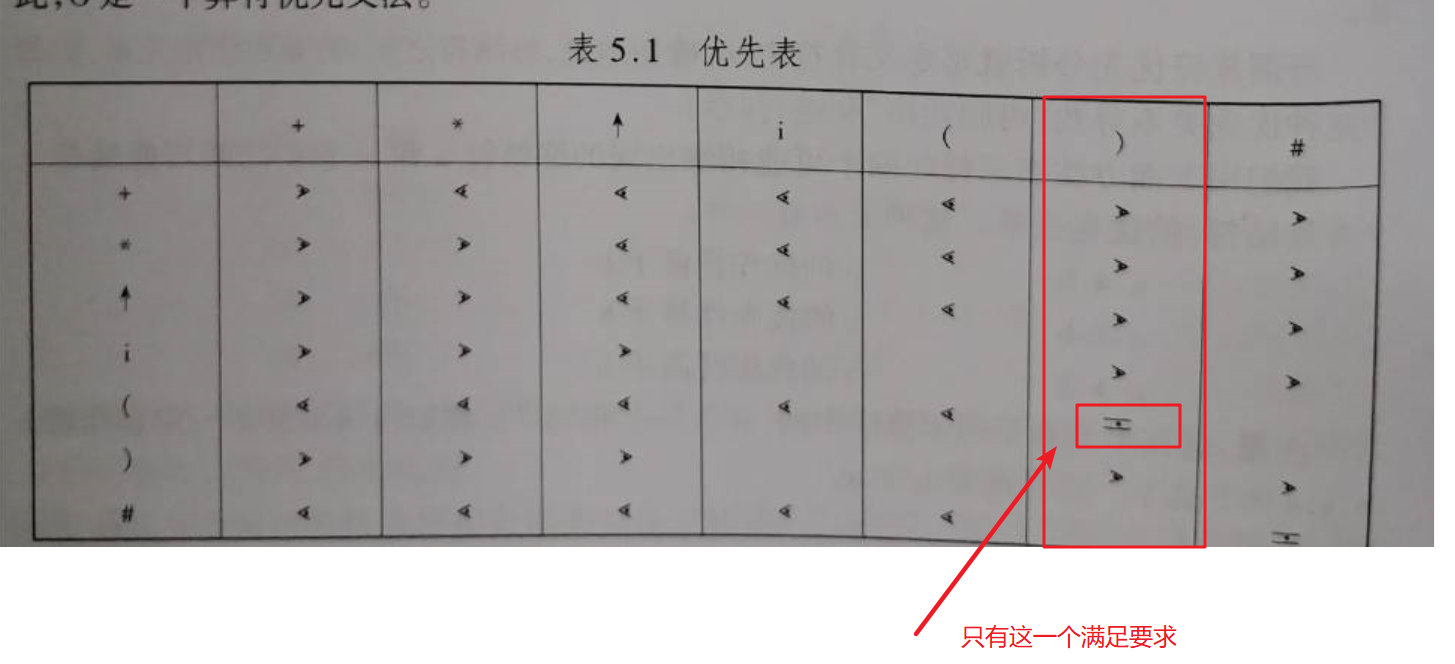但是f(’(’)已经出现过了（作为g(’+’)子树出现的），所以就不用画出来了。
4. 然后就是寻找f(’(’) >=的算符。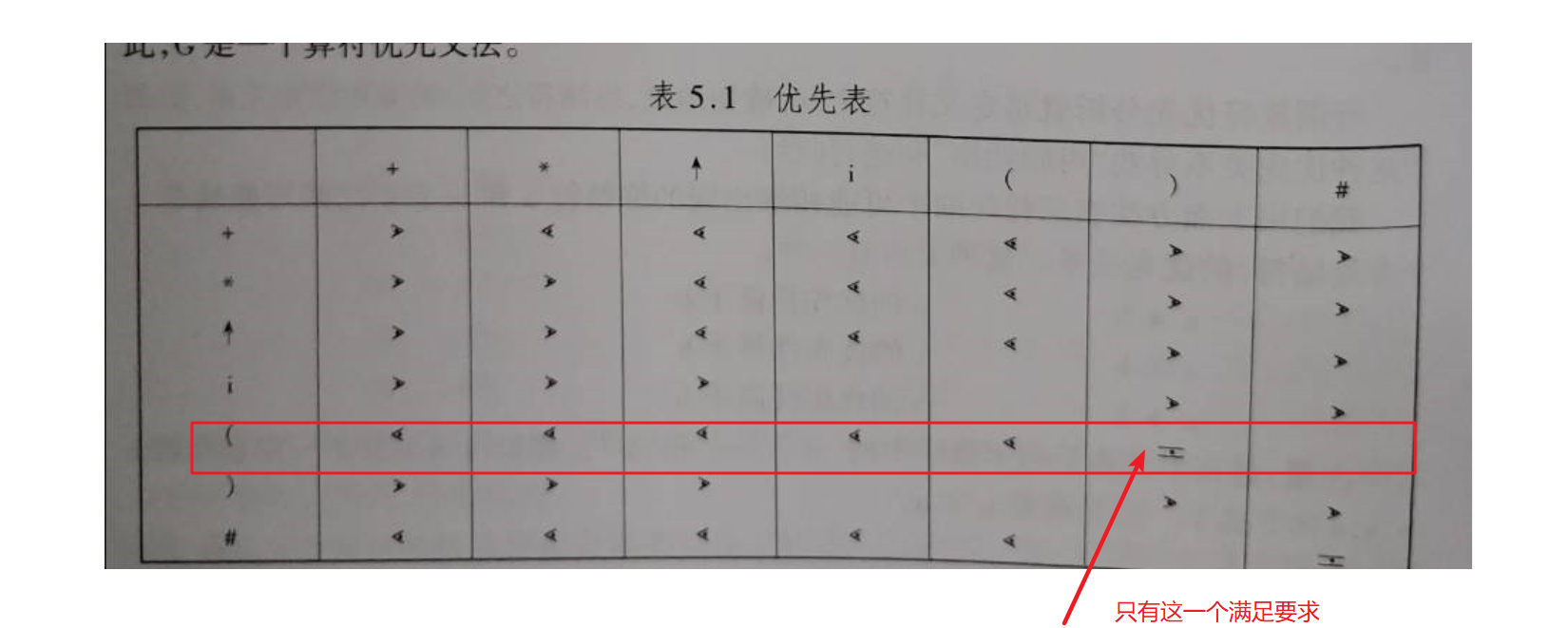但是g(’)’)也已经出现过了，所以这里也不用画出来了。
5. 最后每一个节点我们都已经检查过了，没有可以重新添加的了。也就是趋于稳定了（编译原理里面好多都是这样子的，得使所有都不再变化的时候，算法就算结束了）。我们数一下节点的个数，就是4个，和书本95页给出的答案是一样的。

同理，g(’+’)的树也可以这样画出来。我就给出最后的树，就不一一分析了。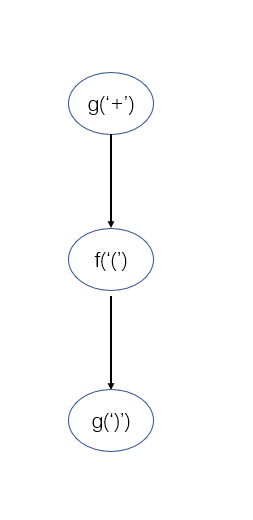# 自己的思考

本质上书本上的画图和这里的画一颗树，都是优先级高的指向优先级低的，所以入度为0的节点，给的优先函数值应大一点，出度为0的，给出的优先函数值应该小一点。

上面提到的这个简单的方法其本质善和我们教材上的方法是一样的。你可以将教材上的方法中的图给截取一部分出来。例如，你截取以g(’+’)作为顶点的树去看，发现就是和我上面画的一样。

还有注意的一点就是：我们求出一组优先函数之后，就可以构造出无数组优先函数。所以，如果你求出来的和参考答案给出的不是一样的，也不一定是你错了。只要你的优先函数之间的关系和参考答案上给出的关系是一样的就可以了。

# 参考资料

构造优先函数的更简单方法_树型构造_潘群娜
这个知网上我找到的一篇关于这个优先函数构造比较简单的方法，如果大家对上面的博客解释的不太清楚的话，可以去知网上看这个原作者写的文章。

展开全文编译原理 算符优先
• 编译原理之求算符优先函数的方法—迭代法 此博文包含图片 (2011-04-26 22:55:38)转载▼ 标签： 编译原理 算符优先函数 迭代法 教育 分类： IT乐园 编译原理之求算符优先函数的方法—迭代法 若已知运算符之间的优先...

原地址

编译原理之求算符优先函数的方法—迭代法 此博文包含图片 (2011-04-26 22:55:38)转载▼ 标签： 编译原理 算符优先函数
迭代法 教育 分类： IT乐园 编译原理之求算符优先函数的方法—迭代法

若已知运算符之间的优先关系，可按如下步骤构造优先函数：
1、对每个运算符a（包括#在内）令f(a)=g(a)=1
2、如果a⋗b且f(a)<=g(b)令f(a)=g(b)+1
3、如果a⋖b且f(a)>=g(b)令g(b)= f(a)+1
4、如果a≐b而f(a) ≠g(b)，令min{f(a),g(b)}=max{f(a),g(b)}
5、重复2~4，直到过程收敛。如果重复过程中有一个值大于2n，则表明不存在算符优先函数。程序实现代码为：

#include <stdio.h>
#include <stdlib.h>
#define MaxSize 100
#define MaxOp 9
struct
{
char ch;  //运算符
int pri;  //优先级
}
lpri[]={{'+',1},{'-',1},{'*',1},{'/',1},{'(',1},{')',1},{'#',1}},
rpri[]={{'+',1},{'-',1},{'*',1},{'/',1},{'(',1},{')',1},{'#',1}};
int f(char op)   //求左运算符op的优先级
{
int i;
for (i=0;i<MaxOp;i++)
if (lpri[i].ch==op) return lpri[i].pri;
}
int g(char op) //求右运算符op的优先级
{
int i;
for (i=0;i<MaxOp;i++)
if (rpri[i].ch==op) return rpri[i].pri;
}
char Precede(char c1,char c2)
{

int i=0,j=0;
static char array={
'>', '>', '<', '<', '<', '>', '>',
'>', '>', '<', '<', '<', '>', '>',
'>', '>', '>', '>', '<', '>', '>',
'>', '>', '>', '>', '<', '>', '>',
'<', '<', '<', '<', '<', '=', '!',
'>', '>', '>', '>', '!', '>', '>',
'<', '<', '<', '<', '<', '!', '='};
switch(c1)
{
case '+' : i=0;break;
case '-' : i=1;break;
case '*' : i=2;break;
case '/' : i=3;break;
case '(' : i=4;break;
case ')' : i=5;break;
case '#' : i=6;break;
}
switch(c2)
{
case '+' : j=0;break;
case '-' : j=1;break;
case '*' : j=2;break;
case '/' : j=3;break;
case '(' : j=4;break;
case ')' : j=5;break;
case '#' : j=6;break;
}
return (array[7*i+j]);
}

void main()
{
int i,j,k=1;
while(k!=0)
{
k=0;
for(i=0;i<7;i++)
{
for(j=0;j<7;j++)
{
if(Precede(lpri[i].ch,rpri[j].ch)=='>'&&f(lpri[i].ch)<=g(rpri[j].ch))
{     lpri[i].pri=rpri[j].pri+1;k=1;}
else if(Precede(lpri[i].ch,rpri[j].ch)=='<'&&f(lpri[i].ch)>=g(rpri[j].ch))
{     rpri[j].pri=lpri[i].pri+1;k=1;}
}
}
}
printf("              ");
for(i=0;i<7;i++)
printf("<",lpri[i].ch);
printf("\n");
printf("入栈优先函数f：");
for(i=0;i<7;i++)
printf("=",lpri[i].pri);
printf("\n");
printf("比较优先函数g：");
for(i=0;i<7;i++)
printf("=",rpri[i].pri);
printf("\n");
}


结果如下：展开全文经验分享
• 优先函数实现表达式计算 左/右 + * ( ) i # + ·> · · ·> · ·> * ·> ·> · ·> · ·> ( · · · ＝ · ) ·> ·> ·> ·> i ·> ·> ·> ...

### 优先函数实现表达式计算

左/右+*()i#
+·>＜·＜··>＜··>
*·>·>＜··>＜··>
(＜·＜·＜·＜·
)·>·>·>·>
i·>·>·>·>
#＜·＜·＜·＜·

由优先表构造优先函数

优先函数+*()i#
f682881
g479291

本程序使用C语言编写
支持整数加减乘除带括号的运算
核心部分和数据结构中利用栈实现表达式运算几乎相同
程序的难点在于优先级＜、＝、＞分类讨论

#include<stdio.h>
#include<stdlib.h>
#include <string.h>
//优先函数
int fplus = 6;
int gplus = 4;

int fmul = 8;
int gmul = 7;

int fleft = 2;
int gleft = 9;

int fright = 8;
int gright = 2;

int fi = 8;
int gi = 9;

int fpound = 1;
int gpound = 1;

char expression;
int flag = 1;

void Error()
{
printf("错误\n");
exit(0);
}

void Input()
{
//char over[] = {'#', '\0'};
printf("请输入表达式，以#结尾：");
gets(expression);
//printf("Input: %s\n",expression);
}

int f(char opt)
{
if(opt == '+' || opt == '-')
{
return fplus;
}
else if (opt == '*' || opt == '/')
{
return fmul;
}
else if (opt == '(')
{
return fleft;
}
else if (opt == ')')
{
return fright;
}
else if (opt == 'i')
{
return fi;
}
else if (opt == '#')
{
return fpound;
}
return -1;
}

int g(char opt)
{
if(opt == '+' || opt == '-')
{
return gplus;
}
else if (opt == '*' || opt == '/')
{
return gmul;
}
else if (opt == '(')
{
return gleft;
}
else if (opt == ')')
{
return gright;
}
else if (opt == 'i')
{
return gi;
}
else if (opt == '#')
{
return gpound;
}
return -1;
}

int In(char num)
{
if(num == '0' ||num == '1' || num == '2' || num == '3' || num == '4' ||
num == '5'|| num == '6' || num == '7' || num == '8' || num == '9')
return 1;
else
return -1;
}

int Operation(int a, char opt, int b)
{
switch(opt)
{
case '+': return a + b;
case '-': return a - b;
case '*': return a * b;
case '/': return a / b;
default : return 0;
}
}

int main()
{
int index_e = 0;	// 字符串下标
int	index_oprt = 0; // 算符栈下标
int index_opnd = 0; // 算量栈下标
int Sn;
char Sp;
int num1;
int num2;
char temp;
Sp = '#';

Input();
//printf("算符栈: %s\n",Sp);
while( flag == 1 )
{
int t = 0;
if( Sp[index_oprt] == '#' && expression[index_e] == '#' )
{
//printf("都为#，结束\n");
flag = 0;
}
else if(Sp[index_oprt] == '(' && expression[index_e] == ')')
{
//printf("读到括号\n");
index_oprt--;
index_e++;
}
else if(In(expression[index_e]) == 1)
{
//printf("读到数字\n");
while(In(expression[index_e]) == 1)
{
temp[t] = expression[index_e];
t++;
index_e++;
}
//printf("temp: %s\n",temp);
//atof()是把字符串转换成double
Sn[index_opnd++] = atof(temp);
//printf("number %d\n",Sn[index_opnd-1]);
//清空temp
for(int m=0; m<100; m++)
temp[m] = '\0';
}
else if(f(Sp[index_oprt]) < g(expression[index_e]))
{
//printf("%d %d\n",f(Sp[index_oprt]),g(expression[index_e]));
//printf("小于\n");
Sp[index_oprt + 1] = expression[index_e];
index_oprt++;
index_e++;
//printf("%c 入栈\n",Sp[index_oprt]);
}
else if(f(Sp[index_oprt]) > g(expression[index_e]))
{
//printf("大于\n");
//出栈两个数
num2 = Sn[index_opnd - 1];
index_opnd--;
num1 = Sn[index_opnd - 1];
index_opnd--;
//算符栈出一个，算完了结果再入算量栈
Sn[index_opnd] = Operation(num1, Sp[index_oprt], num2);
index_opnd++;
index_oprt--;
}
else
{
Error();
}
}
printf("表达式的值为：%d\n", Sn);
return 0;
}


运行截图：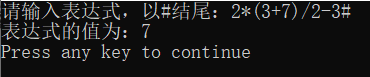展开全文c语言 后端
• 构造优先关系0 目录10 语法分析-自下而上分析110.5 构造优先关系10.5.1 课堂重点10.5.2 测试与作业11 下一章 0 目录 10 语法分析-自下而上分析1 10.5 构造优先关系 10.5.1 课堂重点 10.5.2 ...
• 简单的方法，计算优先函数 手动计算比较麻烦，而且容易出错。 根据定义，用计算机实现，快捷方便，准确可扩充   // 0505优先函数.cpp : 定义控制台应用程序的入口点。 // /* 使用说明： 1、改#define N 的值 2...ini system
• 自底向上分析方法，也称移进-归约分析法。 实现思想： 对输入符号串自左向右进行扫描，并将输入符逐个移入一个栈中，...5.1 自底向上优先分析法概述 5.2 简单优先分析法 5.3 算符优先分析法 ...简单优先分析 最左素短语
• 最易于实现的一种移动归约分析方法，叫做算符优先分析法，而更一般的移动归约分析方法叫做LR分析法，LR分析法可以用作许多自动的语法分析器的生成器。 短语：文法G[S]，αβδ是文法G的一个句型，S=>*αAδ且A=>+...
• 本文关键词：C++ 多态 虚函数表 虚函数指针 动态绑定 概述：C++相对其他面向对象语言来说，之所以灵活、高效。很大程度的占比在于其多态技术和模板技术。C++虚函数表是支撑C++多态的重要技术，它是C++动态绑定...C++ 多态 虚函数 动态绑定
• c++优先队列（自定义比较函数）方式一：struct重载运算符() 方式二：class重载运算符() 方式三：定义函数 方式四：lambda表达式 方式五：function包装lambda表达式 可以使用现成的 less<T>来定义大顶堆 ...c++
• 如果优先函数存在，则可以通过以下三个步骤从优先构造优先函数:(1)对于每个终结符a，令其对应两个符号fa和ga，画一张以所有符号fa和ga为结点的方向图。如果a>.b，则从fa画一条弧至gb如果a，则从gb画一条弧至fa 。...
• c++优先队列官方文档 在c++中优先队列，有自己的库 包含在头文件queue里 template <class T, class Container = vector<T>, class Compare = less<...第三个是比较函数，默认调用 <c++ 队列 数据结构
• 在算符优先分析法中，文法终结符之间的优先关系是用优先矩阵表示的，这样需要占用大量的内存空间，当文法有n个终结符时，就需要（n+1）^2个内存单元，因此，在实际实现中使用优先函数来代替优先矩阵表示优先关系。...
• 函数，返回 实体类型 复杂类型 标量值函数 可组合的 不可组合的 汇总功能 内建功能 尼拉迪奇功能 模型定义的功能 EntityFramework.Functions库可在带有Entity Framework 6.4.0的.NET Standard上运行。 它还可以...C#
• C++中的优先队列实质是一种堆（最大堆或最小堆）队列
• #include &lt;iostream&gt; #include &lt;vector&gt; #include &lt;unordered_map&gt; #include &lt;queue&gt; #include &lt;string&... * 默认使用le...
• Firstvt 找Firstvt的三条规则：如果要找A的Firstvt，A的候选式中出现： A->a…，即以终结符开头，该终结符入Firstvt A->B…，即以非终结符开头，该非终结符...前面是终结符，则终结符入Firstvt 算符优先关系 实例 2.
• ## C++_多态（深入理解虚函数表）

千次阅读 多人点赞 2021-05-08 13:23:17
军人买票时是优先买票 怎么构成多态 并没有构成多态，形参p对象，全部调用了Person类的成员函数。 多态与重写 这时候就需要使用虚函数来构成多态。 梳理一下，多态的条件： 继承类中，需要对虚函数进行重写。 ...多态 指针
• 由于JS编译器的作用，函数声明和变量声明都会被提升，但是一个值得注意的细节是函数会首先被提升，然后才是变量。 提升 变量和函数声明从它们在代码中出现的位置被“移动”到了最上面，这叫变量的提升。分为...变量提升
• //定义一个优先队列默认从大到小排序，因为是优先队列呀 如果我们想从小到大排序就需要自定义排序函数了bool operator > ( Node a,Node b) //这里的参数如果是Node* 并不知道为什么 { return a.num > b.nuc语言 自定义排序
• 最易于实现的一种移动归约分析方法，叫做算符优先分析法，而更一般的移动归约分析方法叫做LR分析法，LR分析法可以用作许多自动的语法分析器的生成器。 短语：文法G[S]，αβδ是文法G的一个句型，S=>*αAδ且A=>+β...
• 使其重写基类的函数f，此时，B类的虚函数表将变成： 这里说明了虚函数表在继承时的另一个行为： 如果子类重写了父类的某些虚函数，在复制父类虚函数表时将会替换那些被子类重写的函数为子类的版本。 虚函数表小结 ...c++ 指针 多态 编程语言
• 在js中函数声明和变量都会被提升,但是函数会被优先提升,然后才是变量下面我们贴出我们的示例代码foo();//1 var foo; function foo() { console.log( 1 ); } foo = function() { console.log( 2 ); }我们使用js引擎...
• 【20200423】编译原理课程课业打卡十八之构造文法算符优先关系&求解输入串算符归约过程 一、课业打卡十八之构造文法算符优先关系&求解输入串算符归约过程 二、知识巩固 1、关于FIRSTVT & LASTVT 2、算符优先关系...编译器
• 关于优先队列priority_queue自定义比较函数用法整理 原来上不了网，写在word里了，代码什么的直接贴过来了，有空整理成高亮的形式。 0.0、首先注意一点，priority_queue没有front()方法，和一般的queue不一样，与...acm
• C++中的虚函数的作用主要是实现了多态的机制。关于多态，简而言之就是用父类型别的指针指向其子类的实例，然后通过父类的指针调用实际子类的成员函数。这种技术可以让父类的指针有“多种形态”，这是一种泛型技术。...
• 有几个例子要展示，以防万一：内联值CREATE FUNCTION MyNS.GetUnshippedOrders() RETURNS TABLE AS RETURN SELECT a.SaleId, a.CustomerID, b.Qty FROM Sales.Sales a INNER JOIN Sales.SaleDetail b ON a....
• ## 算符优先分析法-思路方法在这里

千次阅读 多人点赞 2020-05-16 20:48:28
3.构造并输出算符优先分析，判断是否为算符优先文法，若不是提示无法进行分析； 4.任意输入一个输入串，可得到成功的分析或错误的提示，输出其分析过程或打印语法 分析树。 实验运行结果 算符优先文法的特点： ...编程语言 算法
• 对于派生类的构造 函数，在定义对象时构造函数的执行顺序为： 1：基类的构造函数 2：成员对象的构造函数 3：派生类本身的构造函数 public class IoTest { public static void main(String[] args) throws ...
• import java.util.*; public class DFStu { public static void main(String args... //优先字符，从大到小 //重写比较器 Queue&lt;Character&gt; que = new PriorityQueue( new Comparator&lt;Char......